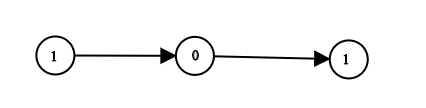Amazon

Amazon

Apple

Bloomberg

Cisco

Citrix

Mathworks

Microsoft

Oracle

Roblox

Snapchat

Yandex

eBay

2020-11-01

## 1290. Convert Binary Number in a Linked List to Integer

### Question:

Given `head` which is a reference node to a singly-linked list. The value of each node in the linked list is either 0 or 1. The linked list holds the binary representation of a number.

Return the decimal value of the number in the linked list.

#### Example 1:``````Input: head = [1,0,1]
Output: 5
Explanation: (101) in base 2 = (5) in base 10
``````

#### Example 2:

``````Input: head = 
Output: 0
``````

#### Example 3:

``````Input: head = 
Output: 1
``````

#### Example 4:

``````Input: head = [1,0,0,1,0,0,1,1,1,0,0,0,0,0,0]
Output: 18880
``````

#### Example 5:

``````Input: head = [0,0]
Output: 0
``````

Constraints:

• The Linked List is not empty.
• Number of nodes will not exceed `30`.
• Each node’s value is either `0` or `1`.

### Solution:

Shift the result to left by 1 and add the node value in to the last binary in result.

``````/**
* Definition for singly-linked list.
* public class ListNode {
*     int val;
*     ListNode next;
*     ListNode() {}
*     ListNode(int val) { this.val = val; }
*     ListNode(int val, ListNode next) { this.val = val; this.next = next; }
* }
*/
class Solution {
public int getDecimalValue(ListNode head) {
int result = 0;
while (head != null) {
result = result << 1;
}
return result;
}
}
``````

Similar solution

``````/**
* Definition for singly-linked list.
* public class ListNode {
*     int val;
*     ListNode next;
*     ListNode() {}
*     ListNode(int val) { this.val = val; }
*     ListNode(int val, ListNode next) { this.val = val; this.next = next; }
* }
*/
class Solution {
public int getDecimalValue(ListNode head) {
ListNode curr = head;
int result = 0;
while(curr != null) {
result = (result << 1) | curr.val;
curr = curr.next;
}
return result;
}
}
``````